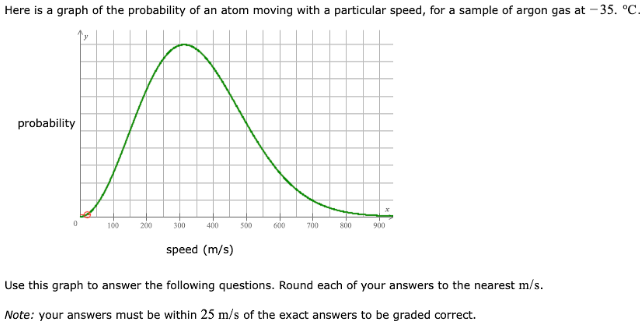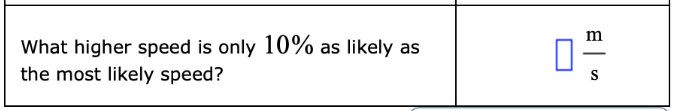# Here is a graph of the probability of an atom moving with a particular speed, for a sample of argon gas at -35. degrees C. Use this graph to answer the following questions. Round each of your answers to the nearest m/s. Note: your answer must be within 25 m/s of the exact answers to be graded correct. What higher speed in only 10% as likely as the most likely speed?